# CBSE Class 10 Mathematics Area Related To Circle Worksheet Set B

Download printable Mathematics Class 10 Worksheets in pdf format, CBSE Class 10 Mathematics Area Related To Circle Worksheet Set B has been prepared as per the latest syllabus and exam pattern issued by CBSE, NCERT and KVS. Also download free pdf Mathematics Class 10 Assignments and practice them daily to get better marks in tests and exams for Grade 10. Free chapter wise worksheets with answers have been designed by Standard 10 teachers as per latest examination pattern

## Worksheet for Class 10 Mathematics Chapter 12 Areas related to Circles

Class 10 Mathematics students should refer to the following printable worksheet in Pdf for Chapter 12 Areas related to Circles in Grade 10. This test paper with questions and solutions for Standard 10 Mathematics will be very useful for tests and exams and help you to score better marks

### Chapter 12 Areas related to Circles Class 10 Mathematics Worksheet Pdf

AREAS RELATED TO CIRCLES

CASE STUDY QUESTION

Q1. John had a farm with many animals like cows, dogs, horses etc. He had sufficient grass land for the cows and horses to graze, One day Three of his horses were tied with 7metre long ropes at the three corners of a triangular lawn having sides 20m, 34m and 42m.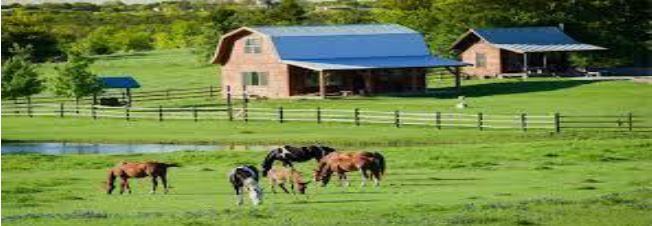(a) Find the a rea of the triangular lawn .

(b) Find the area of the field that can be grazed by the horses.

(c) The area that cannot be grazed by the horses.

Q2. A brooch is a small piece of jewellery which has a pin at the back so it can be fastened on a dress, blouse or coat. Designs of some brooch are shown below. Observe them carefully.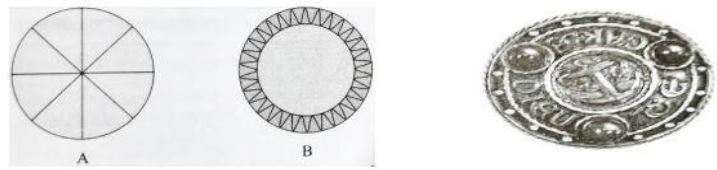Design A: Brooch A is made with silver wire in the form of a circle with diameter 28mm. The wire used for making 4 diameters which divide the circle into 8 equal parts.

Design B: Brooch b is made two colours - Gold and silver. Outer part is made with Gold. The circumference of silver part is 44mm and the gold part is 3mm wide everywhere.

Refer to Design A

1. Find the total length of silver wire required .

2. Find the area of each sector of the brooch is

Refer to Design B

3. Find the circumference of outer part (golden) is

Q.- A wire is looped in the form of a circle of radius 28 cm. It is re-bent into a square form.Determine the length of the side of the square.
Length of the wire = Circumference of the circle
Length of the wire ={ 2 x 22/7 x 28} cm
[Using C = 2πr]
Length of the wire = 176 cm ....(i)
Let the side of the square be x cm. Then,
Perimeter of the square = Length of the wire
4x = 176 [Using (i)]
x = 44 cm
Hence, the  length of the sides of the square is 44 cm.

Q.- A race track is in the form of a ring whose inner circumference is 352 m, and the outer circumference is 396 m. Find the width of the track.
Answer : Let the outer and inner radii of the ring be R metres and r metres respectively. Then,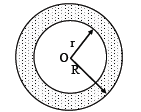2πR = 396 and 2πr = 352
= 2 × 22/7 × R = 396 and 2 × 22/7 × r = 352
=R = 396 × 7/22 × 1/2 and r = 352 × 7/22 × 1/2
=R = 63 m and r = 56 m
Hence, width of the track = (R – r) metres
= (63 – 56) metres = 7 metres
SECTION A:

1. If area of a circle is numerically double its perimeter, then find the radius of the circle.(CBSE 2011)    (4 units)

2. If the area of a sector is 5/18 of the area of the circle, find the angle subtended by the sector at the centre.  (100⁰)

3. If the diameter of a semicircular protractor is 14 cm, then find its perimeter.  (36 cm)

4. A square is inscribed in a circle. What is the ratio of the areas of the circle and the square.   (π : 2)

SECTION B:

5. ABCD is a field in the shape of a trapezium with AD║BC, ∠ABC = 90⁰ and ∠ADC = 60⁰. Four sectors are formed with centres A, B, C and D. The radius at each sector is 14 m. Find the total area of four sectors. (CBSE 2011) (616 m2)

6. Find the number of revolutions made by a circular wheel of area 1.54 cm2 in rolling a distance of 176 cm.(CBSE 2013)   (40)

7. In the given figure three sectors of a circle of radius 7cm , making an angles of 40 ͦ , 60 ͦ , and 80 ͦ at the centre are shaded. Find the area of the shaded region.  (77cm2)8. In fig 2, find the area of the shaded region enclosed between two concentric circles of radii 5cm and 4cm where ∠ AOC =40 0 (CBSE 2018)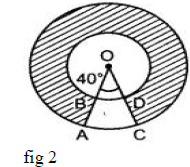SECTION C:

9. The long and short hands of a clock are 6 cm and 4 cm long respectively. Find the sum of the distances travelled by their tips in 24 hours. (Use π = 3.14) (804.57cm)

10. OABC is a rhombus whose three vertices A, B and C lie on a circle with centre O. Find the area of the rhombus, if the area of the circle is 1386 cm2. (220.5√3cm2)

11. The area enclosed between the two concentric circles is 346.5 cm2. The circumference of the inner circle is 88 cm. Find the radius of the outer circle. . (CBSE 2012) (17.5cm)

12. A steel wire when bent in the form of a square encloses an area of 121 sq. cm. If the same wire is bent into the form of a circle, find the area of the circle. (154 cm2)

SECTION D:

13. The diameters of front and rear wheels of a tractor are 80 cm and 2 m respectively. Find the number of revolutions that rear wheel will make in covering a distance in which the front wheel makes 1400 revolutions.  (280)

14. Find the area of the shaded region for fig3. (154.86cm2)

15. Find the area of shaded region in fig.4, where a circle of radius 6 cm has been drawn with vertex O of an equilateral triangle OAB of side 12 cm. (Use π = 3.14 and √3 = 1.73)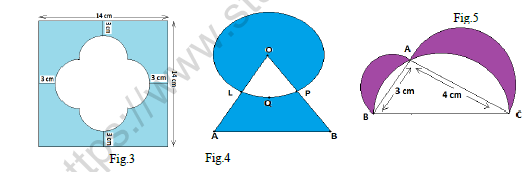16. In fig.5, ΔABC is right angled at A. Semicircles are drawn on AB, AC and BC as diameters. Find the area of shaded region. (CBSE 2014)  (6 sq. units)